Open in App
Not now

# Find determinant of a complex matrix in PyTorch

• Last Updated : 08 Oct, 2021

Linear Algebra module torch.linalg of PyTorch provides the function torch.linalg.det() to compute the determinant of a square(real or complex) matrix. This function also computes the determinant of each square matrix in a batch.

Syntax:

torch.linalg.det(input) –> Tensor

input is an (n, n) matrix or a batch of matrices of size (b, n, n) where b is the batch dimension (number of matrices in a batch). This input must be a tensor. This function returns a tensor of the determinant value/s.

Note: This function supports float, double, cfloat, and cdouble dtypes.

Let’s first look at how to create a complex matrix in PyTorch and then compute the determinant of the matrix. To create a complex matrix in PyTorch, we use 2D Complex Tensors. We can also create a batch of complex matrices. The supported complex dtypes are torch.cfloat and torch.cdouble.

Below are some examples to create a complex matrix (two-dimensional Complex Tensor) and finding their determinant.

Example 1:

Create a 2D complex tensor of size 2×2 by generating random numbers and compute the determinant.

## Python3

 `# import library``import` `torch` `# create 2x2 complex matrix using``# random numbers``mat ``=` `torch.randn(``2``,``2``, dtype ``=` `torch.cfloat)` `# display the matrix``print``(``"Complex Matrix: \n"``, mat)` `# Compute the determinant of Matrix``# using torch.linalg.det()``det ``=` `torch.linalg.det(mat)``print``(``"Determinant: \n"``, det)`

Output: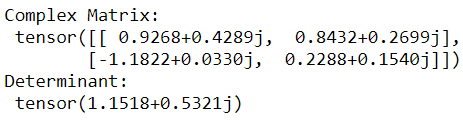Example 2:

Another way to create a complex matrix in PyTorch is to use torch.complex(real, imag). This creates a complex tensor with real and imaginary parts as real and imag. Both real and imag must be float or double and imag must be the same type as real. In the below program we create a 2D complex tensor of size 2×2 and compute its determinant.

## Python3

 `# import library``import` `torch` `# create real parts the complex numbers``real ``=` `torch.tensor([[``1``, ``2``],[``5``,``6``]], dtype``=``torch.float32)` `# create imaginary parts of the complex numbers``imag ``=` `torch.tensor([[``3``, ``4``],[``8``,``9``]], dtype``=``torch.float32)` `# create complex tensor (matrix)``z ``=` `torch.``complex``(real, imag)``print``(``"Complex Matrix: \n"``, z)` `# Compute the determinant of Matrix``# using torch.linalg.det()``det ``=` `torch.linalg.det(z)``print``(``"Determinant: \n"``, det)`

Output: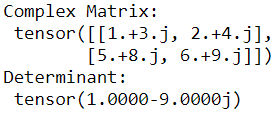Example 3:

Complex tensors can also be created from already existed real tensors. Real tensors of shape (…, 2) can easily be converted into complex tensors using torch.view_as_complex() and their determinant can be computed using torch.linalg.det() method.

## Python3

 `# import library``import` `torch` `# create a real tensor``mat ``=` `torch.randn(``3``,``3``,``2``)` `# display the real tensor``print``(``"Real Tensor: \n"``,mat)` `# convert the above real tensor``# into complex tensor``mat ``=` `torch.view_as_complex(mat)` `# display the complex tensor (matrix)``print``(``"Complex Tensor (Matrix): \n"``, mat)` `# Compute the determinant of Matrix``# using torch.linalg.det()``det ``=` `torch.linalg.det(mat)``print``(``"Determinant: \n"``, det)`

Output: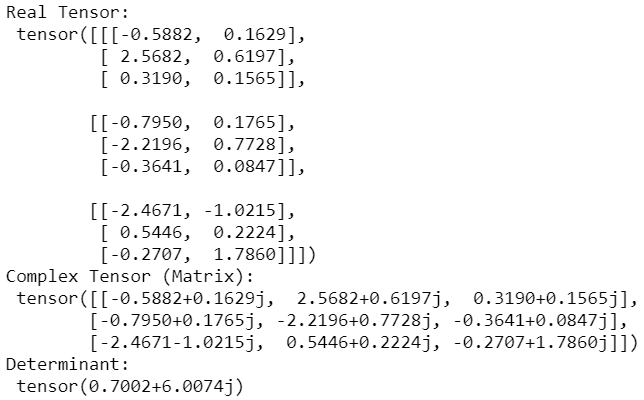Example 4:

Here we create a batch of 3 complex tensors of size 2×2 and compute its determinant.

## Python3

 `# import library``import` `torch` `# create matrix``mat ``=` `torch.randn(``3``,``2``,``2``, dtype ``=` `torch.cfloat)` `# display matrix``print``(``"Batch of Complex Matrices: \n"``, mat)` `# Compute the determinant``det ``=` `torch.linalg.det(mat)``print``(``"Determinant: \n"``, det)`

Output: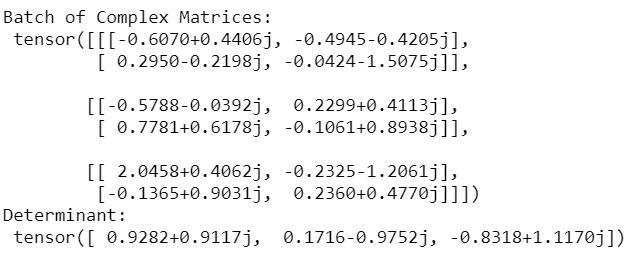Example 5:

Here is another program where we create a batch of 3 complex tensors of size 4×4 and compute the determinant.

## Python3

 `# import library``import` `torch` `# create complex matrix``mat ``=` `torch.randn(``3``,``4``,``4``, dtype ``=` `torch.cfloat)` `# display matrix``print``(``"Batch of Complex Matrices: \n"``, mat)` `# Compute the determinant``det ``=` `torch.linalg.det(mat)``print``(``"Determinant: \n"``, det)`

Output: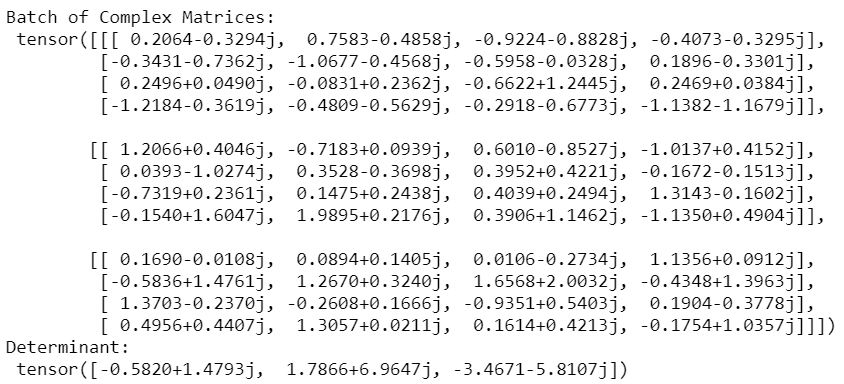My Personal Notes arrow_drop_up